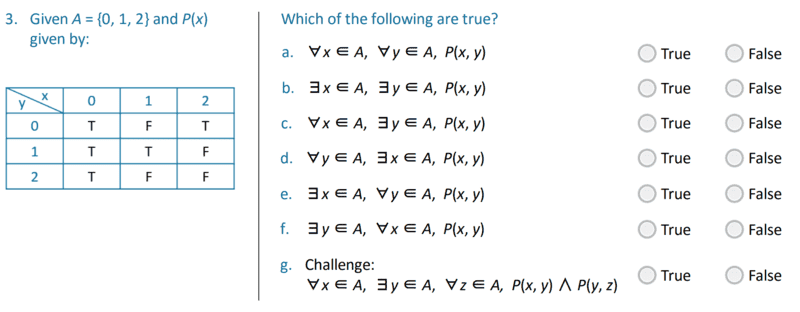# Basic Logic Problem P(x,y)

• WWCY

## Homework Statement

I refer to part G of this little problem:I don't see how to arrive at any conclusion, especially when I can't even see how ##z## comes into play. Assistance in interpreting the problem is appreciated!

## The Attempt at a Solution

I know that the answer for G is "False", which means I have to show that the following is true;
$$\exists x \in A, \ \forall y \in A, \exists z \in A, \ \neg P(x,y) \lor \neg P(y,z)$$

Here's how I have tried to interpret the problem.
$$\neg P(x,y) \lor \neg P(y,z)$$
is true when either or both predicates are false. Looking at a particular ##x## and all combinations of ##y## that come with it (##\exists x \in A, \ \forall y \in A##), none of them are able to make ##P(x,y)## false all the time.

So now I try to look at all ##y## (that are already paired with some ##x##) and try to find some ##z## for each of the ##y## that would cause ##p(z,y)## to always be false. However, I'm not sure where and how to apply the ##z## since it's not defined in the table.

Was this how I am supposed to interpret the problem? Apologies if what I have written is unintelligible as I am finding logic rather confusing.

#### Attachments

the table of truth of P(y,z) is the same as table of truth for P(x,y) with two differences: where x you put y and where y you put z.
that is it is
y 0 1 2
z \
0 T F T
1 T T F
2 T F F​

I see the table I tried to make using standard text editing doesn't look that good but I think you ll understand it. for example it is P(y,z)=T for y=0 and z=0, P(y,z)=F for y=1 and z=0, P(y,z)=T for y=2 and z=0...

•WWCY

## Homework Statement

I refer to part G of this little problem:

View attachment 230498

I don't see how to arrive at any conclusion, especially when I can't even see how ##z## comes into play. Assistance in interpreting the problem is appreciated!

## The Attempt at a Solution

I know that the answer for G is "False", which means I have to show that the following is true;
##\ \exists x \in A, \ \forall y \in A, \exists z \in A, \ \neg P(x,y) \lor \neg P(y,z)##

Here's how I have tried to interpret the problem.
##\ \neg P(x,y) \lor \neg P(y,z)##
...
Don't be fixated on what variable name is used for a particular index (parameter) .

For example: If we set x = 0 and y = 1, then P(x,y) is True. However P(y,x) is False for the same choices of x and y, because this is P(1,0) .

•WWCY and Delta2